Roots and Rational Exponents

Recall thatcan be defined as follows:

1. If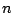is odd, thenis the numbersuch that.

2. Ifis even and, thenis the numbersuch that. (Notice thatis undefined ifis even and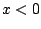.

We can define rational exponents in the following manner:

If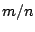is a fraction reduced to lowest terms, then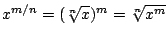,

assuming thatifis even.

Ex 1 Solve the equation.

Sol Squaring both sides gives, and then subtracting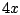from both sides gives. Then factoring gives, so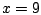or. However,does not check in the original equation, sois the only solution.

Ex 2 Solve the equation.

Sol a Adding 6 to both sides gives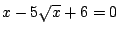, and then factoring gives, so eitheror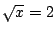and thereforeor.

Sol b Adding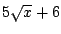to both sides gives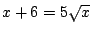, and then squaring both sides gives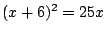; soor.

Pr 1 Simplify the expression.

Pr 2 Solve the equation.

Pr 3 Solve the equation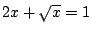.

Pr 4 Solve the following equations:

a). b).

Pr 5 Find all values of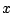for which the equationis valid.

Pr 6 Rewrite the expression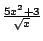as a sum of terms with rational exponents.

Pr 7 Solve the equation.

Pr 8 Solve the equation.

Pr 9 Solve the equation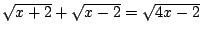.

Pr 10 Solve the equation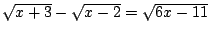.

Pr 11 Solve the equation.